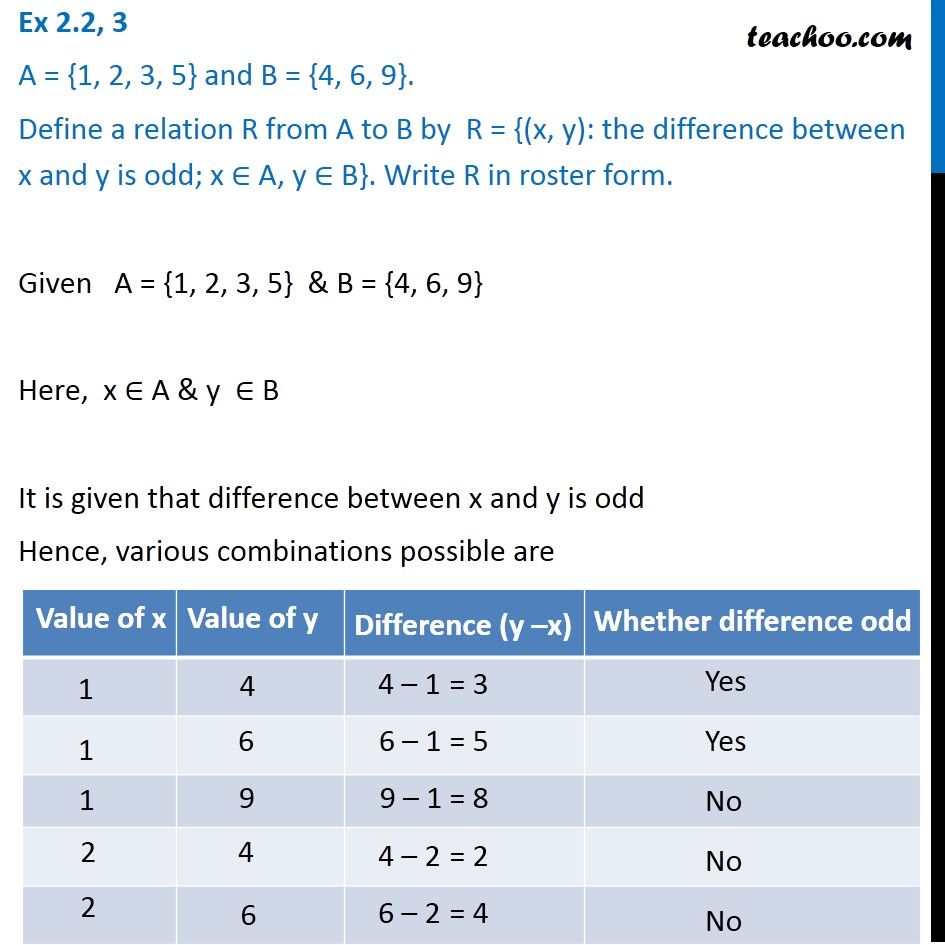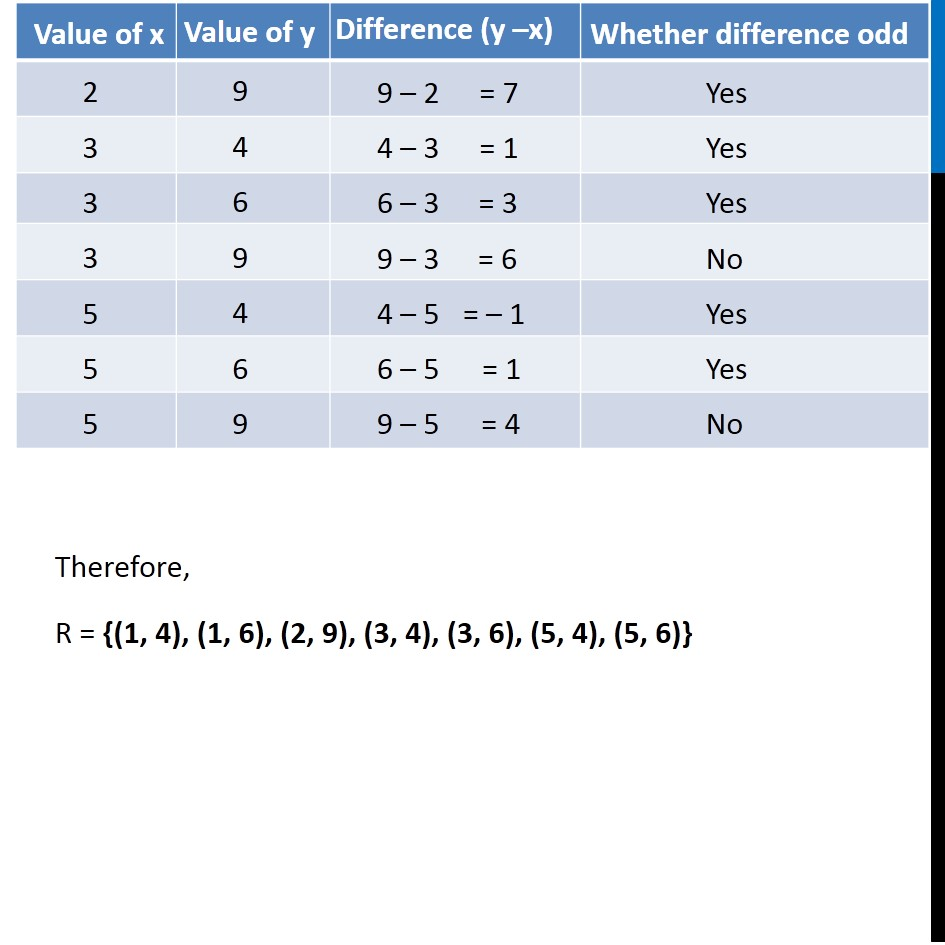Finding Relation - Set-builder form given

Chapter 2 Class 11 Relations and Functions
Concept wiseLearn in your speed, with individual attention - Teachoo Maths 1-on-1 Class

### Transcript

Ex 2.2, 3 A = {1, 2, 3, 5} and B = {4, 6, 9}. Define a relation R from A to B by R = {(x, y): the difference between x and y is odd; x ∈ A, y ∈ B}. Write R in roster form. Given A = {1, 2, 3, 5} & B = {4, 6, 9} Here, x ∈ A & y ∈ B It is given that difference between x and y is odd Hence, various combinations possible are Therefore, R = {(1, 4), (1, 6), (2, 9), (3, 4), (3, 6), (5, 4), (5, 6)}